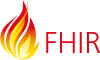# Searching range on numerical value 1e2

Hi Team,
Reading the number searching section Search - FHIR v4.0.1 get confused by the example of the implicit searching range when value=1e2.

``````|[parameter]=1e2 | Values that equal 100, to 1 significant figures precision, so this is actually searching for values in the range [95 ... 105) |
``````

Wouldn’t it be actually in the range [50 … 150) ? because there is only 1 significant digit so the range would be (1 +/- 0.5)e2

ThanksI think you are correct, considering that it also says:
``[parameter]=100` Values that equal 100, to 3 significant figures precision, so this is actually searching for values in the range [99.5 ... 100.5)`
And it doesn’t make sense that the same range is obtained for the same number with two different number of significant figures